American Journal of Astronomy and Astrophysics
Volume 3, Issue 3, May 2015, Pages: 63-69

A Method to Estimate the Cooling Time of Ultra-Relativistic Electrons in Pulsar Wind Nebulae

K. L. I. Gunawardhana1, K. P. S. C. Jayaratne1, J. Adassuriya2

1Department of Physics, University of Colombo, Colombo-03, Sri Lanka

2Astronomy & Space Science Division, Arthur C Clarke Institute for Modern Technologies, Katubedda, Moratuwa, Sri Lanka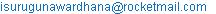(K. L. I. Gunawardhana)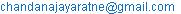(K. P. S. C. Jayaratne)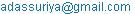(J. Adassuriya)

K. L. I. Gunawardhana, K. P. S. C. Jayaratne, J. Adassuriya. A Method to Estimate the Cooling Time of Ultra-Relativistic Electrons in Pulsar Wind Nebulae. American Journal of Astronomy and Astrophysics. Vol. 3, No. 3, 2015, pp. 63-69. doi: 10.11648/j.ajaa.20150303.16

Abstract: Pulsar is a highly magnetized rotating neutron star. It continuously emits a wind of relativistic electrons and positrons. This wind creates an electron-positron-cloud around the pulsar. This cloud, which is full of relativistic electrons and positrons, is called a Pulsar Wind Nebula (PWN). As of 2014, 33 Pulsar Wind Nebulae (PWNe) have been detected in the TeV energy band. Current understanding is, these TeV photons are produced from up-scattering low-energy photons to high-energies by ultra-relativistic electrons and positrons in PWNe, which is a non-thermal process. This process is known as inverse-Compton scattering. During inverse-Compton scattering, ultra-relativistic electrons lose their energy and cool-down to low-energies. The average time that an ultra-relativistic electron takes to cool-down by inverse-Compton scattering is called the cooling time. Estimation of cooling time is important to understand how the luminosity of a PWN changes with time. This paper describes a statistical method developed for estimating the cooling time of ultra-relativistic electrons in a given PWN. This new method is a model independent technique. Cooling time was estimated as a function of two parameters: k and γ. Here k is the high-energy electron fraction in PWN at a given time and γ is the Average Bulk Lorentz Factor of electrons in the PWN. The estimated cooling time is proportional to k and inversely proportional to γ. The developed method was applied to four PWNe: MSH 15-52, HESS J1420-607, HESS J1825-137 and HESS J1837-069. The estimated cooling times vary between 1.56 kyr to 1000 kyr for MSH 15-52, 13 kyr to 8000 kyr for HESS J1420-607, 21.4 kyr to 10000 kyr for HESS J1825-137 and 22.7 kyr to 15000 kyr for HESS J1837-069.

Keywords: Cooling Time, Inverse-Compton Scattering, Neutron Star, Pulsar, Pulsar Wind Nebula

1. Introduction

Pulsar Wind Nebulae (PWNe) are clouds of relativistic electron and positron. They are continuously powered by the relativistic electron and positron wind that comes from their associated pulsars. Pulsars are highly magnetized rotating neutron stars. In 1934, the existence of neutrons stars was proposed by astrophysicists Walter Baade & Fritz Zwicky (Baade & Zwicky, 1934). Sun like stars will not form neutron stars. Only massive stars (at least 1.5 times larger than our sun) will undergo a supernova explosion and become neutron stars. In 1939, the first equation of state of neutron star was evaluated by J.R. Opppenheimer & G. Volkoff (Oppenheimer & Volkoff, 1939). Then in 1967, F. Pacini (Pacini, 1967) proposed that the electromagnetic waves come from highly magnetized rotating neutron stars. Also he proposed that the Crab nebula was powered by such a star. The first pulsar was discovered by Jocelyn Bell and Anthony Hewish in 1967 who were studying interplanetary scintillation at Cambridge at that time. The pulsar discovered by Bell and Hewish is now called PSR B1919+21: PSR stands for Pulsating source of Radio and B1919+21 indicates the position of the pulsar in the sky. In 1968, Pacini’s proposed theories were confirmed by Bell and Hewish (Hewish & Bell, 1968) using the first discovered pulsar in 1967.

About 1800 pulsars were detected using radio detectors at the end of 2010 (Imagine, 2014). However, only about 70 pulsars were detected using gamma-ray telescopes (Imagine, 2014). More than two-thirds of the currently known pulsars were discovered using the Parkes radio telescope in Australia (Hobbs, 2014). Also the gigantic radio telescope in Puerto Rico, the Green Bank telescopes in America, the Molonglo telescope in Australia and the Jodrell Bank telescope in England were used to detect pulsars.

The most well-known and also a prototypical PWN is "The Crab nebula" (Hester, 2008). The Crab nebula was associated with a supernova (SN) explosion observed in 1054 CE (Stephenson & Green, 2002). In the beginning, a 16th magnitude star embedded in the Crab Nebula was long presumed to be the stellar remnant and central engine (Minkowski, 1942; Pacini, 1967). This was confirmed by after the 33 ms optical and radio pulsations were detected from this star in the late 1960s (Cocke, Disney & Taylor, 1969; Staelin & Reifenstein, 1968) and then these pulsations were shown to be slowing down at a rate of 36 ns per day (Richards & Comella, 1969). So scientists concluded that the Crab nebula contains a rapidly rotating young neutron star (pulsar) formed in the SN explosion of 1054 CE.

At present, 33 TeV PWNe were detected (TeVCat, 2014).Current understanding is these TeV Photon are produced by the inverse-Compton Scattering. During the inverse-Compton Scattering process, low-energy photons become high-energy photons by the ultra-relativistic electrons and positrons in PWNe. Also in the same time ultra-relativistic electrons lose their energy and cool-down to low-energies.

So the process happening inside the PWN is equivalent to a birth & death process. The entering of an electron to PWN can be considered as a birth & the loss of energy of an electron due to inverse-Compton scattering in the PWN can be considered as a death. As the life time in the birth and death process means the time gap between birth and death, the cooling time of ultra-relativistic electrons in PWN means the average time that an ultra-relativistic electron takes to cool-down by inverse-Compton scattering.

In other words, cooling time means the average time of an electron stays in the Pulsar Wind Nebula region, before it emits a TeV gamma-ray photon. The new generation of ground-based Imaging Atmospheric Cherenkov Telescopes (IACTs, e.g: High Energy Stereoscopic System, "HESS") have now begun to detect PWNe in the TeV band. The current structure of the PWNe was defined with as magnetized plasma and which is thought to consist mainly of energetic electrons and positrons (Gaensler & Slane, 2006).

This paper reports a statistical method developed for estimating the inverse-Compton scattering cooling time of ultra-relativistic electrons in PWNe. The cooling time of four PWNe was estimated using this statistical method. This newly developed method is a model independent technique. So, it can be used to estimate the cooling time of ultra-relativistic electrons in any PWN easily. Table I shows the four PWNe and their associated pulsars which were used in this study.

Table I. Selected four TeV PWNe and their associated Pulsars.

 Name of the TeVPWNe Name of the associated Pulsar MSH 15-52 (Aharonian et al., 2005) PSR B1509-58 HESS J1420-607 (Aharonian et al., 2006b) PSR J1420-6048 HESS J1825-137 (Aharonian et al., 2006c) PSR J1826-1334 HESS J1837-069 (Aharonian et al., 2006a) PSR J1838-0655

2. The Theory and Method

In this Section, we introduce a new statistical technique to estimate the cooling time of ultra-relativistic electrons in PWN. The rate of loss pulsar’s rotational kinetic energy (energy output of the pulsar) is called spin-down luminosity (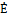).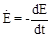(1)

Spin-down luminosity changes with time. Following Pacini & Salvati (Pacini & Salvati, 1973), spin-down luminosity as a function of time can be written as follows: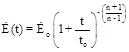(2)

where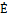o =(t=0), to is the spin-down timescale and n is the braking index. Following Mayer et al. (Mayer at el., 2013; Equation (3)), spin-down timescale (to) is defined as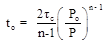(3)

where

tc = Characteristic age of the pulsar

Po = Initial period of the pulsar

P = Current period of the pulsar

Characteristic age is a rough estimate of the real age of the pulsar. Following Mayer et al. (Mayer at el., 2013; Equation (4)), it is defined as,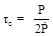(4)

Here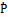denotes the time derivative of the rotational period of the Pulsar.

Let’s define a new term called inverse-Compton scattering probability. Inverse-Compton scattering probability is the probability of creation of a TeV photon by inverse-Compton scattering of an ultra-relativistic electron in unit time and it is denoted as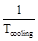, where Tcooling is the cooling time.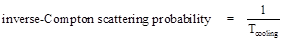(5)

In other words,is called the inverse-Compton scattering frequency. This frequency is denoted as "b". So the relationship between b &can be written as follows.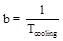(6)

In order to find b, one has to find an expression for the number electrons exist in PWN at time t. Figure I shows the block diagram of structure of the Pulsar and PWN system.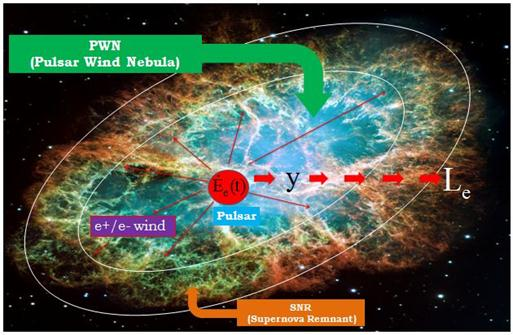Figure I. Structure of the Pulsar and Pulsar Wind Nebula system (Adapted from: hubblesite.org).

Here y denotes the number electrons exist in PWN at a given time t. The technique was used to obtain an expression for y is similar to a water tank that drain water while filling in. If we know the incoming rate of water to the tank and out going rate of water from the tank, we can find the amount of water at time t using a first order differential equation. In this study, the incoming rate is the number of electrons enter to the PWN per unit time and out going rate is the number of electrons cool-down per unit time in PWN. The rate of change of y can be written as;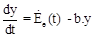(7)

where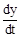= rate of change of the number electrons exist at time "t" in the PWNe(t) = rate of electrons coming in to the PWN

b.y = rate of electrons cool-down in the PWN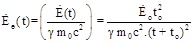(8)

In equation (8),(t) is the total energy comes to PWN. It is divided by the energy per electron (γ m0c2) to get the number of electrons come to PWN per unit time.o = initial spin down luminosity of the pulsar

to = spin down time scale of the pulsar

γ = average bulk Lorentz factor,

mo = rest mass of the electron= 0.511 MeV/c2= 0.511 x 10-6TeV/c2

c = velocity of light

γ moc2= 0.511 x 10-6γ TeV

b = probability that an high energy electron go through inverse-Compton scattering per unit time (inverse-Compton scattering frequency)

y = number of electrons exist at time t in the PWN

In other words,e (t) denotes the birth rate and b.y denotes the death rate of the process. An expression for y can be obtained solving the differential equation 7,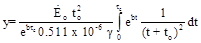(9)

This y is not a measurable quantity. However, luminosity of a PWN (Le) in a given time is a measurable parameter. Luminosity due to inverse-Compton scattered photons is equal to the number of high energy electrons exist at a given time (k.y) times the probability of one of these electrons go through inverse-Compton scattering (b).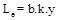(10)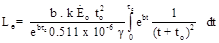(11)

where Le is number of TeV photons per second.

The above equation has 2 unknown parameters except b. They are k and γ. Here k is the high energy electron fraction in PWN and γ is the Average Bulk Lorentz Factor of electrons which are coming from the pulsar wind. Theo and to belong to the pulsar properties and Le is a PWN property. The exact k and γ values of the four PWNe, which were used in this paper, are not estimated yet. Therefore the "b" values were found for a group of k and γ values.

3. Analysis and Results

3.1. Pulsar Properties

The position of each of four pulsars with coordinates of right ascension and declination were given in Table II.

Table II. Position of the four Pulsars.

 PWNe Pulsar Right ascension Declination MSH 15-52 PSR B1509-58 15h 13m 55.52s −59° 08′ 08.80″ HESS J1420-607 PSR J1420-6048 14h 20m 08.20s −60° 48′ 17.20″ HESS J1825-137 PSR J1826-1334 18h 25m 41.00s −13° 50′ 20.00″ HESS J1837-069 PSR J1838-0655 18h 37m 38.40s −06° 57′ 00.00″

The rotational period (P) and its time derivative (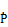) of all four pulsars were found from the ATNF pulsar database and those values were given in Table III.

Table III. P and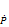values of the four Pulsars.

 PWNe Pulsar P (Period) [s] MSH 15-52 PSR B1509-58 0.15125125831483 1.5314675176E-12 HESS J1420-607 PSR J1420-6048 0.06817987659000 8.3167E-14 HESS J1825-137 PSR J1826-1334 0.10148679420760 7.5251738E-14 HESS J1837-069 PSR J1838-0655 0.07049824396900 4.925E-14

The characteristic ages of the four pulsars were given in Table IV.

Table IV. Characteristic ages of the four Pulsars.

 PWNe Pulsar tc [kyr] MSH 15-52 PSR B1509-58 1.56 HESS J1420-607 PSR J1420-6048 13.00 HESS J1825-137 PSR J1826-1334 21.40 HESS J1837-069 PSR J1838-0655 22.70

The initial period values of above all four pulsars were taken from Mayer et al. 2013; Page 6, TABLE II. The following Table V shows the Po values for all four pulsars.

Table V. Initial period (Po) values of the four Pulsars.

 PWNe Pulsar Po[s] MSH 15-52 PSR B1509-58 0.0389 HESS J1420-607 PSR J1420-6048 0.0332 HESS J1825-137 PSR J1826-1334 0.0260 HESS J1837-069 PSR J1838-0655 0.0307

The spin-down timescale (to) values of the four Pulsars were given in the Table VI.

Table VI. Spin-down timescale (to) values of the four Pulsars.

 PWNe Pulsar to[kyr] MSH 15-52 PSR B1509-58 0.10 HESS J1420-607 PSR J1420-6048 3.08 HESS J1825-137 PSR J1826-1334 1.40 HESS J1837-069 PSR J1838-0655 4.30

The following Table VII shows that the current rate of loss of rotational kinetic energies (current spin-down luminosity, at t= tc ) of the four pulsars.

Table VII. Current spin-down luminosity values (at t= tc ) of four Pulsars.

 PWNe Pulsar[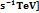t=tc [kyr] MSH 15-52 PSR B1509-58 1.123471682 * 1037 1.56 HESS J1420-607 PSR J1420-6048 0.624150934 * 1037 13.0 HESS J1825-137 PSR J1826-1334 0.174762261 * 1037 21.4 HESS J1837-069 PSR J1838-0655 0.343283013 * 1037 22.7

The initial spin-down luminosity (rate of loss of rotational kinetic energy) values for the four pulsars were calculated using their current rate of spin-down luminosity values. Table VIII shows the calculated initial spin-down luminosities. Here the braking index (n) of all four pulsars was taken as 3.

Table VIII. Initial spin-down luminosity values (at t=0) of four Pulsars.

 PWNe Pulsar [ o [ MSH 15-52 PSR B1509-58 1.123471682 * 1037 3.095838567*1039 HESS J1420-607 PSR J1420-6048 0.624150934 * 1037 1.701219220*1038 HESS J1825-137 PSR J1826-1334 0.174762261 * 1037 4.635123151*1038 HESS J1837-069 PSR J1838-0655 0.343283013 * 1037 1.353452225*1038

3.2. Photon Flux and Luminosity Values of the Four PWNe

Table IX shows the Photon flux values and TeV Luminosity values of the four PWNe. Here photon flux means, the number of photon emitting across 1cm-2 of the area of the PWN per second (s-1) & luminosity means the number of photon emitting from the whole surface of the PWN per second (s-1).

Table IX. Photon flux and Luminosity (Le) values of the 4 PWNe.

 PWNe D (distance from the erath) [kpc] [Photon flux] ( Luminosity(s-1) {Le values} MSH 15-52 5.81 4.063721902 * 10-9 1.641320937 * 1037 HESS J1420-607 7.65 4.217512693 * 10-9 2.953224983 * 1037 HESS J1825-137 4.12 41.106580530 * 10-9 8.348780603 * 1037 HESS J1837-069 6.60 8.573337232 * 10-9 4.468430867 * 1037

3.3. The k-γ Phase Space of the Cooling Time of the Four PWNe

The k-γ phase space graphs of cooling time are shown in Figure II a-d. In these graphs Tcooling is the cooling time with unit of kyr (thousand years) and cooling time values are illustrated using colored squares. The color bar on the right side of each graph indicates the corresponding Tcooling value for each color. The x axis of each graph is the Average Bulk Lorentz factor (γ) of electrons in the PWN and the y axis of each graph is the high energy electron fraction (k) in PWN. Also z axis (colored squares) is the cooling time (Tcooling). The white color in every plot (Figure II a-d) indicates the region of Tcooling < 0. Cooling time cannot be a negative value. Therefore, this region gives the forbidden space for k and γ. The blue color diagonal in every plot (Figure II a-d) indicates the characteristic age of the associated pulsar of each PWN. The Characteristic age of a pulsar is calculated using the Equation (2) and the calculated values are given in the Table IV. The cooling time should be greater than the characteristic age of the associated Pulsar (pulsar’s characteristic age range 1-20 kyr) of a PWN (Mattana et al., 2009). So that the lower limit of the cooling time is equal to the characteristic age of the pulsar. The upper limit of the cooling time depends on the k and γ values of the PWN and it is found by using the following k-γ phase space graphs of the cooling time.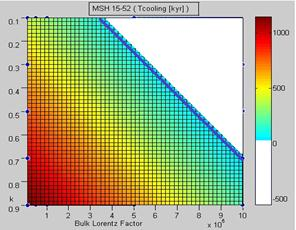Figure II a. k-γ phase space of the cooling time for MSH 15-52.

This is the k-γ phase space of the cooling time for MSH 15-52. In this, value of blue color line is 1.56 kyr. Here k varies from 0.1 to 0.9 and γ varies from 1 x 104 to 1.0 x 106. The estimated range for cooling time is 1.56 – 1000 kyr.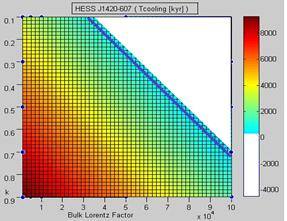Figure II b. k-γ phase space of the cooling time for HESS J1420-607.

This is the k-γ phase space of the cooling time for HESS J1420-607. In this, value of blue color line is 13 kyr. Here k varies from 0.1 to 0.9 and γ varies from 1 x 103 to 1.0 x 105. The estimated range for cooling time is 13 – 8000 kyr.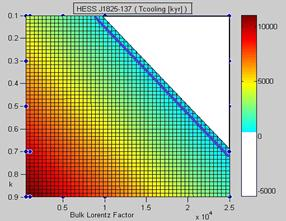Figure II c. k-γ phase space of the cooling time for HESS J1825-137.

This is the k-γ phase space of the cooling time for HESS J1825-137. In this, value of blue color line is 21.4 kyr. Here k varies from 0.1 to 0.9 and γ varies from 5 x 102 to 2.5 x 104. The estimated range for cooling time is 21.4 – 10000 kyr.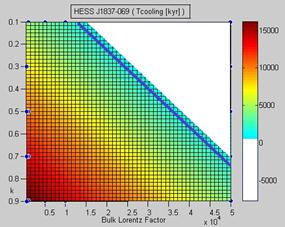Figure II d. k-γ phase space of the cooling time for HESS J1837-069.

This is the k-γ phase space of the cooling time for HESS J1837-069. In this, value of blue color line is 21.4 kyr. Here k varies from 0.1 to 0.9 and γ varies from 5 x 102 to 2.5 x 104. The estimated range for cooling time is 22.7 – 15000 kyr.

Table X. Ranges of the cooling time vary of the four PWNe.

 PWNe Ranges of the cooling time [kyr] Ranges of the k Ranges of the γ MSH 15-52 01.56 - 1000 0.1 – 0.9 1 x 104 – 1.0 x 106 HESS J1420-607 13.00 - 8000 0.1 – 0.9 1 x 103 – 1.0 x 105 HESS J1825-137 21.40 - 10000 0.1 – 0.9 5 x 102 – 2.5 x 104 HESS J1837-069 22.70 - 15000 0.1 – 0.9 5 x 102 – 5.0 x 104

4. Discussion

We found that the cooling time depends on k and γ. Here k is the high energy electron fraction in PWN. It means the ratio of number of high energy electrons in PWN to total number of electrons in PWN. So k is fraction which should lie between zero and one. To our knowledge, exact k and γ for the four PWNe used here are not estimated yet. So we used five different k values (k = 0.1, 0.3, 0.5, 0.7, 0.9) for all four PWNe. However, one can use any value for k, but it should be in the range between zero and one because k is a fraction. Also one can use more than five values for k. If someone estimates the exact value for k for a PWNe then that value can be applied directly for k.

Here γ is the Average Bulk Lorentz Factor of electrons in PWN. According to Thomson regime, photons with energy ~ 1 TeV are produced by electrons with Lorentz factor ~ 0.1 – 3 x 107(Mattana et al., 2009). Also γ depends on the characteristic age of the pulsar of the PWN. So we used four different ranges of γ values for four PWNe. The used γ values vary between 1 x 104 to 1 x 106 for MSH 15-52, 1 x 103 to 1 x 105 for HESS J1420-607, 5 x 102 to 2.5 x 104 for HESS J1825-137 and 5 x 102 to 5 x 104 for HESS J1837-069.

Using the average γ for a given PWN can be identified as a draw back in our calculation. One could improve the calculation using an energy dependent γ. However, choosing an energy dependent γ could increase the complexity of the calculation, and may need to use a numerical technique.

Another assumption we did in our calculation is, b is a constant. However, b could be a function of the seed photon density and energy. Abeysekara & Linnemann (Abeysekara & Linnemann, 2015) argued that the seed photon density for inverse-Compton scattering could be a strong function of pulsar properties.

5. Conclusions

According to the inverse-Compton in the Thomson regime energy losses, the cooling time can be written as (Rybicki & Lightman, 1979).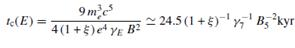(12)

For typical nebula magnetic field intensities B ~ 1–100 μG (Mattana et al., 2009). Equation (12) indicates that the cooling time of γ-ray radiating particles. For example, for B=10 μG, t ~ 8-250 kyr (Mattana et al., 2009).

Another formula for the inverse-Compton cooling time valid in Thompson regime can be written as (Araudo et al., 2009).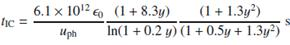(13)

Our technique can be applied to any PWN with a detectable pulsar. The estimated ranges of the cooling time using the new method here, are also in thousand year (kyr) scale (Table X). So these results verify that the new method develop here can be used to estimate the cooling time of ultra-relativistic electrons in PWN. If someone estimates accurately the k and γ values for a PWN, then one can find the exact "b" value from(14)

and then proceed to find the exact cooling time by applying it to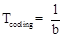(15)

Acknowledgement

We would like to give our special thanks to Dr. A. Udara Abeysekara at Department of Physics and Astronomy, University of Utah, for guiding our work, giving us constructive feedback, and for the encouragement to publish this work.

References

1. Abeysekara, A. U. & Linnemann, J.T. (2015) Experimental Constraints on γ–ray Pulsar Gap Models and the Pulsar GeV to Pulsar Wind Nebula TeV Connection. ApJ. 804. p. 25.
2. Aharonian, F., Akhperjanian, A. G., Aye, K.-M., et al. (2005) A&A. 435 (17).
3. Aharonian, F., Akhperjanian, A. G., Bazer-Bachi, A. R., et al. (2006a) ApJ. 636. p. 777.
4. Aharonian, F., Akhperjanian, A. G., Bazer-Bachi, A. R., et al. (2006b) A&A. 456. p. 245.
5. Aharonian, F., Akhperjanian, A. G., Bazer-Bachi, A. R., et al. (2006c) A&A. 460. p. 365.
6. Araudo, A. T., Bosch-Ramon, V. & Romero, G. E. (2009) High-energy emission from jet-clump interactions in microquasars. A&A. 503. p. 673-681.
7. Baade, W. & Zwicky, F. (1934) On super-novae. Proc. Nat. Acad. Sci. 20. p. 254-259.
8. Cocke, W. S., Disney, M. J. & Taylor, D. J. (1969) Nature. 221. p. 525-527.
9. Gaensler, B. M. & Slane, P. O. (2006) ARA&A. 44. p. 17.
10. Hester, J. Jeff (2008) The Crab Nebula: An Astrophysical Chimera. ARAA. 46 (1). p. 127–155.
11. Hewish, A., Bell, S. J., Pilkington, J. D. H., Scott, P. F. & Collins, R. A. (1968) Observation of a rapidly pulsating radio source. Nature. 217. p. 709-713.
12. Hobbs, M. (2014) An Introduction to Pulsars. [Online] Available from:http://www.atnf.csiro.au/outreach/education/everyone/pulsars/index.html[Accessed: 24th March 2014]
13. Imagine (2014) IMAGINE THE UNIVERSE!. [Online] Available from:http://imagine.gsfc.nasa.gov/science/objects/pulsars2.html[Accessed: 25th March 2014]
14. Mattana, F., Falanga, M., Gotz, D., et al. (2009) THE EVOLUTION OF THE γ - AND X-RAY LUMINOSITIES OF PULSAR WIND NEBULAE. ApJ. 694. P. 12-17.
15. Mayer, M., Brucker, J., Holler, M., Jung, I., Valerius, K. &Stegmann, C. (2013) Predictimg the X-ray flux of evolved pulsar wind nebulae based on VHE γ ray observations. arXiv:1202.1455v3
16. Minkowski, R. (1942) ApJ. 96. p.199-213.
17. Oppenheimer, J. R. & Volkoff, G. (1939) On massive neutron cores. Phys. Rev. 55. p. 374-381.
18. Pacini, F. (1967) Energy emission from a neutron star. Nature. 216. p. 567-568.
19. Pacini, F. & Salvati, M. (1973) ApJ. 186 p. 249.
20. Richards, D. W. & Comella, J. M. (1969) Nature. 222. p. 551-552.
21. Rybicki, G. W. & Lightman, A.P. (1979) Radiative Processes in Astrophysics. (New York: Wiley)
22. Staelin, D. H. & Refenstein, E. C. (1968) Science 162. p. 1481-1483.
23. Stephenson, F. R. & Green, D. A. (2002) Historical Supernovae and Their Remnants. (Oxford: Clarendon Press)
24. TeVCat (2014) Welcome to TeVCat!. [Online] Available from:http://tevcat.uchicago.edu/[Accessed: 29th March 2014].

 Contents 1. 2. 3. 3.1. 3.2. 3.3. 4. 5.
Article ToolsAbstractPDF(477K)